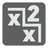The Good Radix
Elementary+
English RU

In mathematical numeral systems, the radix or base is the number of unique digits (including zero) that a positional numeral system uses to represent numbers. For example: in the decimal system, the radix is ten. This is because it has ten digits from 0 through 9. In a system with a radix of 13, there would be 13 digits. For example: a string of digits such as 398 denotes the decimal number 3 * 13^2 + 9 * 13^1 + 8 * 13^0. For the systems with a radix more than 10, we will use capital Latin symbols from A to Z, where A = 10, B = 11 ...

You are given some number n written as a string with the radix equal to k (1 < k < 37). We know that our number is divisible by (k - 1) without a remainder. You should find the minimal possible k, if it exists, or return 0.

For example:... should be greater than 8.
If k == 9, then n = 17 in the decimal system and 17 % 8 == 1. The wrong radix.
If k == 10, then n = 18 in the decimal system and 18 % 9 == 0. We found the answer.

You should be an authorized user in order to see the full description and start solving this mission.